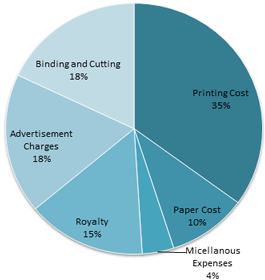The percentage expenses on various items during book production and sale.(a) The cental angle for the sector on “Paper-Cost” is :
[A]22.5°
[B]36°
[C]16°
[D]54.8°

36°
100%=360°
∴1%=360°/100
∴10%=360°*10/100=36°

(b) If the ‘Printing -Cost’ is 17500 RS. The royalty paid is :
[A]6300 Rs.
[B]7500 Rs.
[C]8750 Rs.
[D]3150 Rs.

7500 Rs.
35% total cost = Rs. 17500
∴15% of total cost =$Rs. \frac{17500\times 15}{35} = Rs. 7500$

(c) If the “miscellaneous expenses” are Rs. 6000. How much more are “binding and cutting charges” than “Royalty”?
[A]Rs. 10500
[B]Rs. 4500
[C]Rs. 6000
[D]Rs. 5500

Rs. 4500
Difference in per cent cost of ‘binding and cutting charges’ and ‘rotalty’ = (18-15)%= 3%
Now, 4% of total cost = Rs. 6000
∴ 3% of total cost =$Rs. \frac{6000\times 3}{4}= Rs. 4500$

(d) The central angle corresponding to the sector on “Printing Cost” is more than that of “Acvertisement Charges” by:
[A]54.8°
[B]61.2°
[C]72°
[D]60°

61.2°
Difference in per cent expensses on printing cost and advertisement charges = (35 – 18)% = 17%
Now, 1% = 3.6%
∴ 17% = 3.6°$\times$17=61.2°

(e) The “Paper Cost” is approximately what percent of “Printing Cost”?
[A]32.5%
[B]30%
[C]20.3%
[D]28.6%

28.6%
The required per cent =$\frac{10\times 100}{35}$= 28.6% (approx)